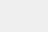# 积分中值定理的推广与积分函数公式研究

## 1. 积分中值定理的基础形式$$\frac{1}{b-a}\int_a^b f(x)dx = f(c).$$

## 2. 积分中值定理的推广

### 2.1 高阶导数的情况

$$\frac{f(b) – f(a)}{b-a} = f'(c).$$

### 2.2 向量值函数的情况

$$\int_a^b \mathbf{F}(t) \cdot \mathbf{F}'(t) dt = \mathbf{F}(b) \cdot \mathbf{F}(c) – \mathbf{F}(a) \cdot \mathbf{F}(c).$$

## 3. 积分函数公式

1. 幂函数的积分公式：

• $\int x^n dx = \frac{1}{n+1}x^{n+1} + C$，其中$n$不等于$-1$。
• $\int \frac{1}{x} dx = \ln|x| + C$。
2. 三角函数的积分公式：

• $\int \sin x dx = -\cos x + C$。
• $\int \cos x dx = \sin x + C$。
• $\int \tan x dx = \ln|\sec x| + C$。
• $\int \cot x dx = \ln|\sin x| + C$。
3. 指数函数与对数函数的积分公式：

• $\int e^x dx = e^x + C$。
• $\int \ln x dx = x\ln x – x + C$。

## 4. 总结